# While Loop in C++

## While Loop in C++ with Examples:

In this article, I am going to discuss While Loop in C++ Language with definitions, syntax, flow charts, and examples. Please read our previous articles, where we give a brief introduction to Loops in C++.

##### What is While Loop in C++ Language?

A loop is nothing but executes a block of statements repeatedly as long as the condition is true. How many times it will repeat means as long as the given condition is true. When the condition fails, it will terminate the loop execution.

A while loop is used for executing a statement repeatedly until a given condition returns false. Here, statements may be a single statement or a block of statements. The condition may be any expression, and true is any nonzero value. The loop iterates while the condition is true. Once you see the syntax and flow chart, then you will get more clarity of the while loop.

##### While loop syntax in C++:

We will show you their skeleton and also the flow chart. Let us look at the syntax of the while loop. While loop checks the condition written inside ‘( )’ is true or not. If the condition is true, then statements written in the body of the while loop i.e., inside the braces { } are executed again condition is checked the process repeats until the condition is false.

while (<condition>){
// process
}

This is the syntax of the while loop. In parenthesis, we will give the condition of termination of the loop. In the curly braces, we write the set of statements that we want to execute repeatedly. While we are working with a while loop first, we need to check the condition, if the condition is true then control will pass within the body and if the condition is false, then the control will pass outside the body.

When we are working with an iteration statement after execution of the body control will be passed back to the condition until the condition becomes false. If the condition is not false then we will get an infinite loop. It is something similar to the if condition, just condition, and statements, but the flow is different from the if condition. How it is different lets us understand through the flow-chart.

##### Flowchart of While Loop in C++:

The following diagram shows the flow chart of the while loop.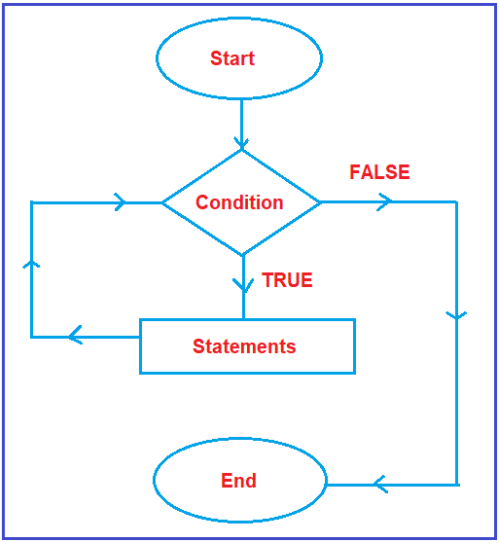The flow chart will start. The start is represented by the oval symbol. Then it will check the condition. As discussed earlier, every condition is having two outputs i.e. true and false. If it is true what will happen and it is false what will happen, we need to check.

Suppose, the condition is true, then what all statements defined inside the block (within the while loop block) will execute. After execution of statements, will it end? NO, it will not end. After execution of statements, once again it will go and check the condition. It will repeat the same process as long as the given condition is true. Suppose, the condition is false, then it will come to end. This is the execution flow of a while loop.

##### Print Numbers from 1 to n using while loop:

Let us first look at the flowchart: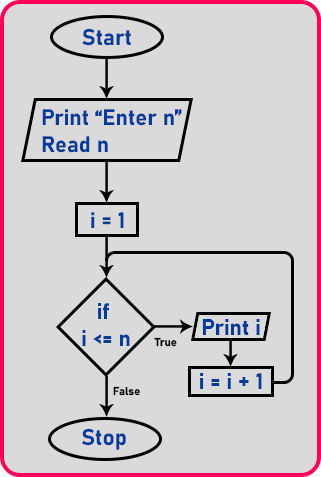1. Step 1: First, we will take the input as far as we want to print the number.
2. Step 2: So, we want to print the number to a certain point. For that, we need a counter, so here we have ‘i’ as a counter. And we have initialized ‘i’ to 1. So ‘i’ starts from one onwards.
3. Step 3: Then the condition will check ‘if (i <= n)’ (n is the input). If the condition is true then print the value of ‘i’ and modify ‘i’ as ‘i = i + 1’.
4. Step 4: Again, the condition will be checked, if it is true then repeat the same steps which we discussed above, or if it is false the loop will be stopped and control will come out of the loop.

Suppose we want to print numbers from 1 to 4, the value of n is ‘4’ and then we initialized the counter as ‘i = 1’ and then check the condition as, if (i <= n), means if (1 <= 4), true, so it will print ‘i’ and then increment ‘i’ as ‘i = 1 + 1’: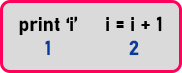Again, check for the condition If (i <= n), means if (2 <= 4), true, so it will print ‘i’ and then increment ‘i’ as ‘i = 2 + 1’: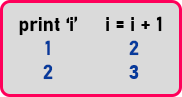Again, if (i <= n), means if (3 <= 4), true, so it will print ‘i’ and then increment ‘i’ as ‘i = 3 + 1’: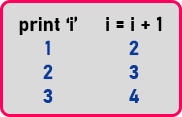Again, if (i <= n), means if (4 <= 4), true, so it will print ‘i’ and then increment ‘i’ as ‘i = 4 + 1’: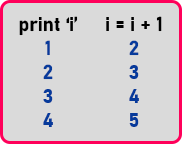Now, if (5 <= 4), false, so here while loop will be stopped. Now let us write code for that.

##### Program to Print numbers from 1 to n using while loop in C++ language:
```#include <iostream>
using namespace std;
int main()
{
int n, i = 1;
cout << "Enter number:" << endl;
cin >> n;
cout << endl;

while (i <= n)
{
cout << i << endl;
i++;
}

return 0;
}
```
###### Output: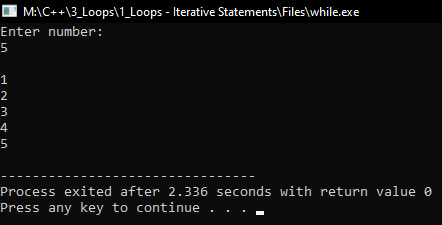##### Program to print one statement for n time using while loop:
```#include <iostream>
using namespace std;
int main()
{
int n, i = 1;
cout << "Enter number:" << endl;
cin >> n;
cout << endl;
while (i <= n)
{
cout << "Hello C++" << endl;
i++;
}
return 0;
}
```
###### Output: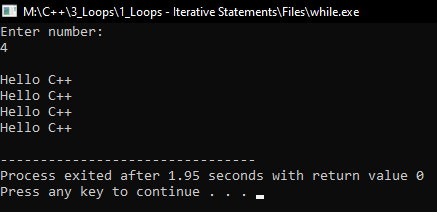In the above example, the variable i is initialized with value 1 and then it has been tested for the condition. If the condition returns true then the statements inside the body of the while loop are executed else control comes out of the loop. The value of i is incremented using the ++ operator then it has been tested again for the loop condition.

##### What is the pre-checking process or entry-controlled loop?

The pre-checking process means before the evaluation of the statement block conditional part will be executed. When we are working with a while loop always pre-checking process will occur. The loop in which before executing the body of the loop if the condition is tested first then it is called an entry-controlled loop.

While loop is an example of the entry-controlled loop because in while loop before executing the body, first, the condition is evaluated, if the condition is true then the body will be executed otherwise the body will be skipped.

##### Nested While Loop in C++:

Writing while loop inside another while loop is called nested while loop or you can say defining one while loop inside another while loop is called nested while loop. That is why nested loops are also called “loop inside the loop”. There can be any number of loops inside one another with any of the three combinations depending on the complexity of the given problem. In implementation when we need to repeat the loop body itself n number of times then we need to go for nested loops.

##### Syntax Nested While Loop in C++:

Following is the syntax to use the nested while loop in C++.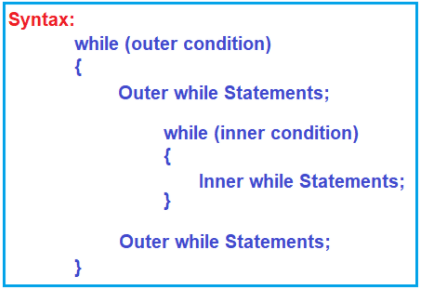Note: In the nested while loop, the number of iterations will be equal to the number of iterations in the outer loop multiplied by the number of iterations in the inner loop which is almost the same as nested for loop. Nested while loops are mostly used for making various pattern programs in C++ like number patterns or shape patterns.

##### Execution Flow of Nested While Loop in C++ Programming Language:

The outer while loop executes based on the outer condition and the inner while loop executes based on the inner condition. Now let us understand how the nested while loop executes. First, it will check the outer loop condition and if the outer loop condition fails, then it will terminate the loop.

Suppose if the outer loop condition is true, then it will come inside, first, it will print the outer loop statements which are there before the inner loop. Then it will check the inner loop condition. If the inner while condition is true, then the control move inside and executes the inner while loop statements. After execution of inner while loop statements, again, it will check the inner while loop condition because it is a loop and as long as the condition is true, it will repeat this process. Once the inner while loop condition fails, then the control moves outside and executes the statements which are present after the inner while loop. Once it executes then, again it will go and check the outer while loop condition. And if it is true, then it will again execute the same process.

So, when the loop will terminate means when the outer while loop condition becomes false.

##### Flow Chart of Nested While Loop:

Please have a look at the following diagram, which represents the flow chart of the nested while loop.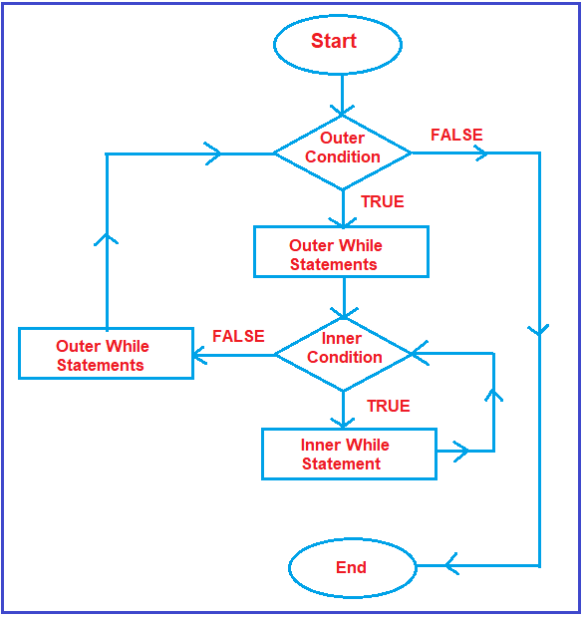The flow will start and first, it will check the outer while loop condition. And if the outer while loop condition failed, then it will come to end. Suppose, the outer loop condition is true, then it will first execute the outer while loop statements if any. After execution of Outer while loop statements, it will check the inner while loop condition. For the inner while loop condition, it will also check for true and false. Suppose, the inner while loop condition is true, then inner while loop statements are executed. After executing the inner while loop statements, again, it will check the inner while loop condition, and this inner loop execution process will repeat as long as the inner while loop condition is true. If the inner while loop condition is false, then the remaining outer loop statements are executed. Once, the outer loop statements are executed, then again, it will come and check the outer while condition. This is the flow of the nested while loop.

##### Example: WAP to print the following format.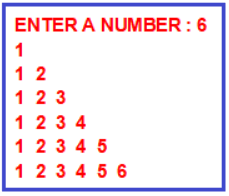###### Program:
```#include <iostream>
using namespace std;
int main()
{
int i = 1, n, in;
cout << "ENTER  A NUMBER ";
cin >> n;

while (i <= n)
{
cout << "\n";
in = 1;
while (in <= i)
{
cout << in;
in = in + 1;
}
i = i + 1;
}
return 0;
}
```
##### Example: WAP to print the following format: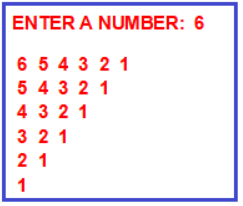###### Program:
```#include <iostream>
using namespace std;
int main()
{
int i, n, dn;
cout << "ENTER  A NUMBER ";
cin >> n;
i = n;
while (i >= 1)
{
cout << "\n";
dn = i;
while (dn >= 1)
{
cout << dn;
dn = dn - 1;
}
i = i - 1;
}
return 0;
}
```

Note: The While Loop is popularly known as pre-tested loops. Here before entering into C++ statements, conditions will be tested and then only control will go to the block of statements in the while loop. It is an example of a conditional loop whereas for loop is an example of a counter loop.

While is used when you do not know how many times you have to repeat, so repeat WHILE condition is true. for is used when you know FOR how many times you have to repeat. In while loop Block of statements are repeatedly executed as long as the condition is true.

#### Use case scenarios of while loop:

##### Program to reverse a number.

Here I want to reverse the number 1024. The reversal number is 4201. How we can achieve this reverse of a number using a while loop?

##### Here is the Logic.

Here n=1024 to extract the last digit of the number to the first place we need to perform modulo of number 1024 by 10, which gives remainder 4.

Remainder =n%10 means 1024%10 gives 4.

Reverse number= Reverse number *10+remainder //here reverse number the first digit becomes 4
N=n/10; now divide the number 1024 now after division N=102

###### Repeat the same step

Remainder=N%10 which gives 2

Reverse number=Reverse number*10+remainder (4*10+2) means 42 in this step two number was reversed.
N=N/10 now divide the number 102 by 10 now after division N=10;

###### Repeat the same step

Remainder=N%10 remainder is zero

Reverse number= Reverse number *10+remainder (42*10+0) this step given reverse number has 420
N=N/10 now N becomes 1 after division

Note already three digits got reverses successfully

###### Repeat the same step

Remainder =N%10 1%10 means remainder 1

Reverse number=Reversenumber*10+ Remainder (420*10+1) 4201 successfully reversed
N=N/10 1/10 is 0;

So, to exit the condition N should not be equal to zero. This is the best use case of the while loop as we don’t know how many times, I need to execute the loop however I know till what condition I need to execute the loop.

```#include <iostream>
using namespace std;
int main()
{
int N;
cout << "enter the number which you would like to reverse" << endl;
cin >> N;
int remainder = 0, reversenumber = 0;
while (N != 0)
{
remainder = N % 10;
reversenumber = reversenumber * 10 + remainder;
N /= 10;
}
cout << "reversenumber is\t" << reversenumber << endl;
return 0;
}
```
###### Output: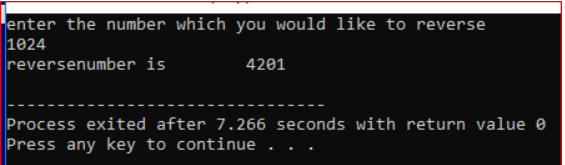In the next article, I am going to discuss Do While Loop in C++ Language with examples. Here, in this article, I try to explain the While Loop in C++ Language with examples. I hope you enjoy this While Loop in C++ Language with Examples article. I would like to have your feedback. Please post your feedback, question, or comments about this While Loop in C++ Language with Examples article.